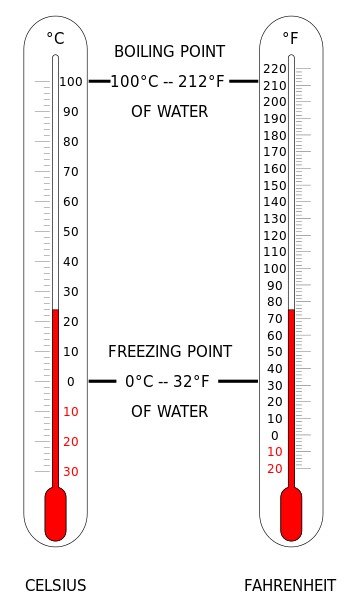# Celsius = Fahrenheit?In order to convert a Fahrenheit temperature $F$ to a Celsius temperature $C,$ we use the following formula:

$C = \frac{5}{9}(F - 32)$

What is the numerical value at which both the Celsius and Fahrenheit scale indicate the same temperature?

×